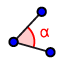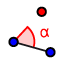# GEOMETRY Notes 1.4: Measure and Classify Angles

Try these problems while you are waiting for class to begin! :)

## #1 and 2

#1) Find the midpoint of . Show the midpoint on the graph, and write the name and coordinates of the point.

#2) If C is the midpoint of , plot D on the graph. What are the coordinates of D?

#3) What is the midpoint of given P(-2, 3p) and Q(4n, p)?

﻿Wait for the class, please. :)
﻿RAY: A ray has one endpoint and extends indefinitely in one direction. OPPOSITE RAYS: two rays with a common endpoint that form a straight line.

## #4

#4) Use the applet! a) First, move around . Change the color by selecting the ray, and then selecting the color tool (this purple square will only appear when you select the ray.)

b) Why would it be confusing to call this ray ?

b) Draw another ray using the ray tool. (Select, and then RAY) c) Move the two rays so that they seem to be OPPOSITE RAYS. d) Now, apply the Drag Test. (Drag Test: if you drag the figure around, do you still have the figure you meant to draw, or does it change?)

## #5

#5) Can you draw a ray that is OPPOSITE to my ray, so that it will pass the Drag Test? Use different colors for your rays.
#6) Use colors to complete #6 on your PRINTED NOTES.
﻿An ANGLE consists of two different rays with the same endpoint. The rays are SIDES and the endpoint is the VERTEX.
#7) a) Use the applet! Drag my angle around. b) Draw an angle by drawing two rays with a common endpoint. c) Draw an angle using the angle tool.Add rays. d) Draw an angle using the angle with a given measure tool.Add rays.
Do #8 on your PRINTED NOTES.

## #9

#8) Sometimes you will not see the entire rays that make the angle sides-- for example, if the angle is part of a triangle. In the applet, lengthen and shorten the segment sides of the angle-- do longer sides mean a larger angle? Name this angle in 3 different ways.

MEASURING ANGLES ON GEOGEBRA!

## #9

#9) Measure the angle shown with the class, and then check your answer. Now spend some time changing the angle, and measuring it. Be sure to measure small and large angles, and also to use both protractors.
CLASSIFYING ANGLES

## #10

#10) Write an equation involving the angles in the applet.

## #11)

﻿BREAKOUT ROOMS: Do #14 and 15 with your partner on your PRINTED NOTES. Next, do the "Check Yourself"-- I'll post the answers to the check yourself, so you can see if you're ready to take your quiz.